# What should be the length of an organ pipe, open at both ends, if the fundamental...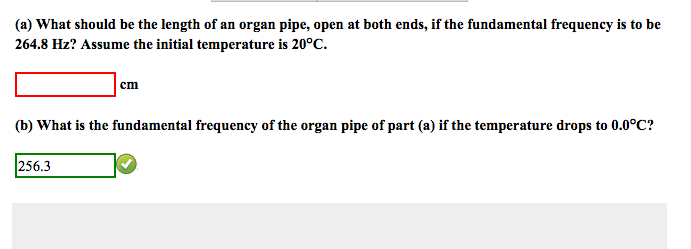What should be the length of an organ pipe, open at both ends, if the fundamental frequency is to be 264.8 Hz? Assume the initial temperature is 20 degree C. What is the fundamental frequency of the organ pipe of part (a) if the temperature drops to 0.0 degree C?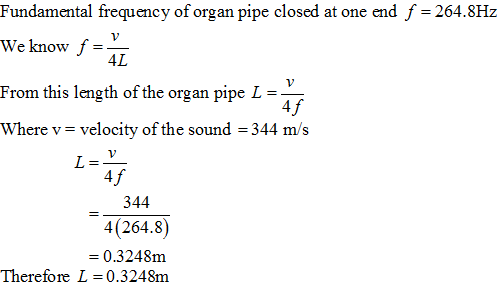#### Earn Coin

Coins can be redeemed for fabulous gifts.

Similar Homework Help Questions
• ### Organ pipe A with both ends open has a fundamental frequency of 320.0 Hz. The third...

Organ pipe A with both ends open has a fundamental frequency of 320.0 Hz. The third harmonic of organ pipe B with one end open has the same frequency as the second harmonic of pipe A. Assume a speed of sound of 343 m/s. What is the length of Pipe A? What is the length of Pipe B?

• ### An open organ pipe (i.e., a pipe open at both ends) of length L has a...

An open organ pipe (i.e., a pipe open at both ends) of length L has a fundamental frequency f. If the organ pipe is cut in half, what is the new fundamental frequency?

• ### The fundamental of an organ pipe that is closed at one end and open at the...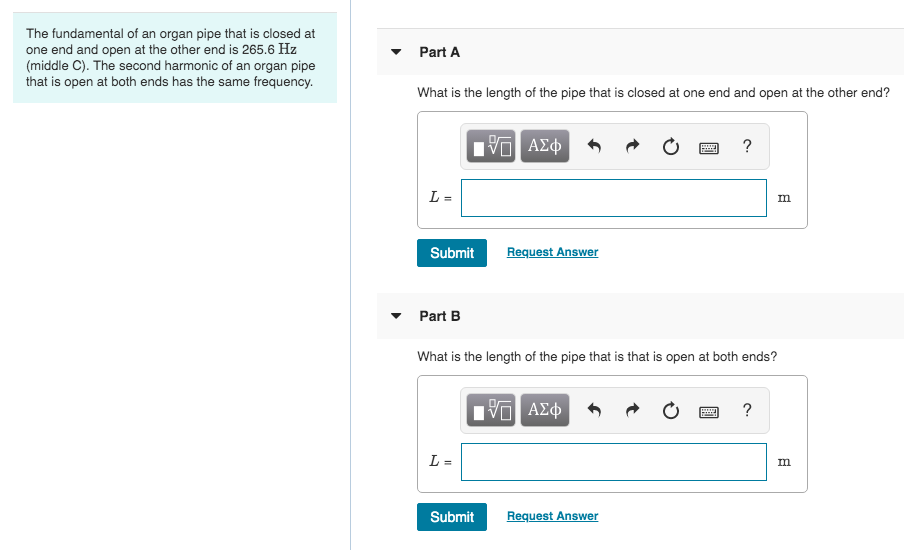The fundamental of an organ pipe that is closed at one end and open at the other end is 265.6 Hz (middle C). The second harmonic of an organ pipe that is open at both ends has the same frequency. Part A What is the length of the pipe that is closed at one end and open at the other end? PO AJĄ O O ? Submit Request Answer Part B What is the length of the pipe that is...

• ### A certain organ pipe, open at both ends, produces a fundamental frequency of 271 Hz in...

A certain organ pipe, open at both ends, produces a fundamental frequency of 271 Hz in air. If the pipe is filled with helium at the same temperature, what fundamental frequency f He will it produce? Take the molar mass of air to be 28.8 g/mol and the molar mass of helium to be 4.00g/mol .

• ### A certain organ pipe, open at both ends, produces a fundamental frequency of 297 Hz in...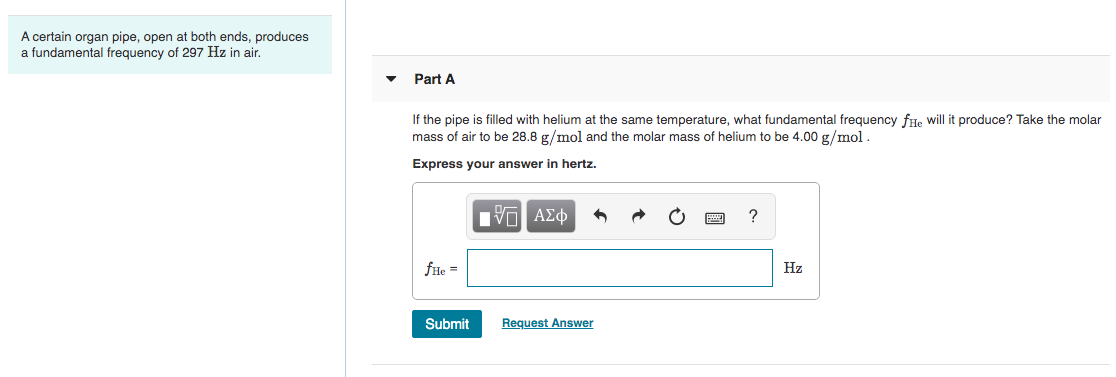A certain organ pipe, open at both ends, produces a fundamental frequency of 297 Hz in air. Part A If the pipe is filled with helium at the same temperature, what fundamental frequency fHe will it produce? Take the molar mass of air to be 28.8 g/mol and the molar mass of helium to be 4.00 g/mol. Express your answer in hertz. V ALD O O ? file Submit Request Answer

• ### Part A: A certain organ pipe, open at both ends, produces a fundamental frequency of 300...

Part A: A certain organ pipe, open at both ends, produces a fundamental frequency of 300 Hz in air. If the pipe is filled with helium at the same temperature, what fundamental frequency fHe will it produce? Take the molar mass of air to be 28.8 g/mol and the molar mass of helium to be 4.00 g/mol. I calculated this correctly to be 879 Hz, but I am not sure about the next part. Now consider a pipe that is...

• ### A certain organ pipe, open at both ends, produces a fundamental frequency of 290 Hz in...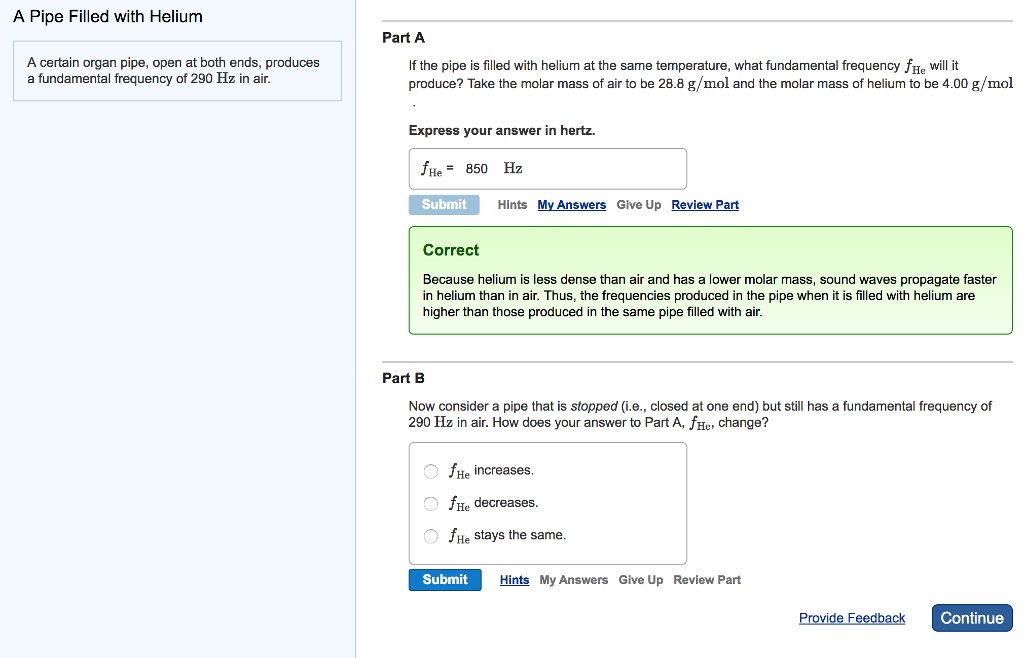A certain organ pipe, open at both ends, produces a fundamental frequency of 290 Hz in air. If the pipe is filled with helium at the same temperature, what fundamental frequency f_He will it produce? Take the molar mass of air to be 28.8 g/mol and the molar mass of helium to be 4.00 g/mol Express your answer in hertz. Now consider a pipe that is stopped (i.e., closed at one end) but still has a fundamental frequency of 290...

• ### 2B.5 An organ pipe open at both ends has a fundamental frequency of 440 Hz (concert...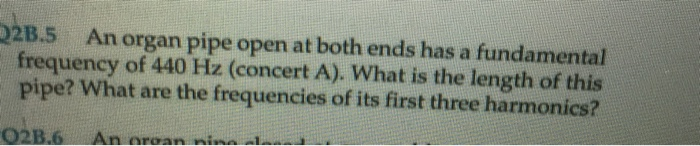2B.5 An organ pipe open at both ends has a fundamental frequency of 440 Hz (concert A). What is the length of this pipe? What are the frequencies of its first three harmonics? 02B.6 An aroan nina

• ### (a) What length of pipe open at both ends has a fundamental frequency of 3.75 102...

(a) What length of pipe open at both ends has a fundamental frequency of 3.75 102 Hz? Find the first overtone. lpipe = .457 Incorrect: m fovertone = 750 Correct: Hz (b) If the one end of this pipe is now closed, what is the new fundamental frequency? Find the first overtone. ffundamental = 750 Incorrect: Hz fovertone = 615 Incorrect: Hz (c) If the pipe is open at one end only, how many harmonics are possible in the normal...

• ### A certain organ pipe, open at both ends, produces a fundamental frequency of 280Hz in air. If the pipe is filled with...

A certain organ pipe, open at both ends, produces a fundamental frequency of 280Hz in air. If the pipe is filled with helium at the same temperature, what fundamental frequency f_He will it produce? Take the molar mass of air to be 28.8g/mol and the molar mass of helium to be 4.00g/mol . I have already tried 877Hz and it is incorrect!